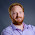## Saturday, 2 May 2015

### Performance of getitem

I've previously posted about how scanning is slow, and I stand by that.  But I've recently discovered that in some situations, it can be less slow than using getitem.

I was doing a code review of some old code and found that it was using \$scan in a situation where I thought you'd usually use getitem, and wondered why someone would have done it this way.  But before I replaced it, I wanted to check the performance to see what difference I would be making by changing it, and I was surprised by the results!

The code was designed to check if a variable matched one of a reasonably large number of items.  For this example, if stuck with 10 items...

if ( temp = "ONE" | temp = "TWO" | temp = "THREE" | temp = "FOUR" | temp = "FIVE" | temp = "SIX" | temp = "SEVEN" | temp = "EIGHT" | temp = "NINE" | temp = "TEN" )
;testing condition
endif

I could have used a line continuation marker, but you get the idea.

What the developer had done is replaced this set of conditions with a single \$scan, like this...

list = "|ONE|TWO|THREE|FOUR|FIVE|SIX|SEVEN|EIGHT|NINE|TEN|"

if ( \$scan(list,"|%%temp%%%|") > 0 )
;testing condition
endif

Note that this is not a Uniface list, a "bar" or "pipe" character has been used as the delimiter - this is placed at the beginning and end of the value to ensure it is not found as a sub-part of another longer value.  It This takes up a lot less space, and is perfectly readable, but I was concerned about performance.

To test this, I wanted to make sure it was fair, so I decided to always check both the first and the last item in the list in each iteration.  I also wanted to test in a few different ways, and I came up with 4...

1) Set of conditions

temp = "ONE"
if ( temp = "ONE" | temp = "TWO" | temp = "THREE" | temp = "FOUR" | temp = "FIVE" | temp = "SIX" | temp = "SEVEN" | temp = "EIGHT" | temp = "NINE" | temp = "TEN" )
;testing condition
endif
temp = "TEN"
if ( temp = "ONE" | temp = "TWO" | temp = "THREE" | temp = "FOUR" | temp = "FIVE" | temp = "SIX" | temp = "SEVEN" | temp = "EIGHT" | temp = "NINE" | temp = "TEN" )
;testing condition
endif

2) \$scan a bar delimited string

list = "|ONE|TWO|THREE|FOUR|FIVE|SIX|SEVEN|EIGHT|NINE|TEN|"

if ( \$scan(list,"|ONE|") > 0 )
;testing condition
endif
list = "|ONE|TWO|THREE|FOUR|FIVE|SIX|SEVEN|EIGHT|NINE|TEN|"
if ( \$scan(list,"|TEN|") > 0 )
;testing condition
endif

3) getitem/id a Uniface list

list = "ONE·;TWO·;THREE·;FOUR·;FIVE·;SIX·;SEVEN·;EIGHT·;NINE·;TEN"
getitem/id temp,list,"ONE"
if ( \$status > 0 )
;testing condition
endif
list = "ONE·;TWO·;THREE·;FOUR·;FIVE·;SIX·;SEVEN·;EIGHT·;NINE·;TEN"
getitem/id temp,list,"TEN"
if ( \$status > 0 )
;testing condition
endif

4) \$item a Uniface list

list = "ONE·;TWO·;THREE·;FOUR·;FIVE·;SIX·;SEVEN·;EIGHT·;NINE·;TEN"
if ( \$item("ONE",list) != "" )
;testing condition
endif
list = "ONE·;TWO·;THREE·;FOUR·;FIVE·;SIX·;SEVEN·;EIGHT·;NINE·;TEN"
if ( \$item("TEN",list) != "" )
;testing condition
endif

I wasn't really sure what I was expecting, but I thought the \$scan would be the worst performance.  I tested over 2,000,000 iterations, and here's what I got...

1) Set of conditions: 37.30, 36.95, 37.83 = 37.36 secs
2) \$scan a bar delimited string: 21.76, 21.97, 21.24 = 21.66 secs
3) getitem/id a Uniface list: 24.46, 24.22, 24.89 = 24.52 secs
4) \$item a Uniface list: 22.65, 23.25, 23.47 = 23.12 secs

So the slowest was the set of conditions.  I didn't test it, but knowing that if statements shortcut I figure that if the item was always passing the first condition it would be quick, but because half of my test items were only passing the last condition, it would have to check each of the conditions in the set before it passed.

Although there wasn't a big difference, what surprised me is that the getitem/id and \$item were actually slower than the \$scan in this case.  I then remembered back to a conversation on the Uniface-L mailing list, which talked about how Uniface handles lists in the background.  The description there indicates that an array is built in the background, which means there is an upfront cost for calling getitem/id (or \$item) once, but then if you're looping through it is much quicker to access the rest, because the array can be used.  However, because I'm rebuilding the list each time, that means the array needs to be rebuilt each time.

This means that actually \$scan can be used to improve the performance when checking that an item is in a list, as long as you're only checking this list once and it's not going to be re-used.  I expect there to be a point at which the number of times the list is re-used means that using getitem/id (or \$item) would become better for performance.

Also, I've not tested different lengths of lists.  However, given the results and my reasoning for why the results ended up this way, I would have thought extending the list would simply emphasize the results.

Summary:  Checking if an item is in a list can be done a number of ways, and if it's being done a lot of times, performance can be eeked out by using a \$scan, surprisingly!

1.2.